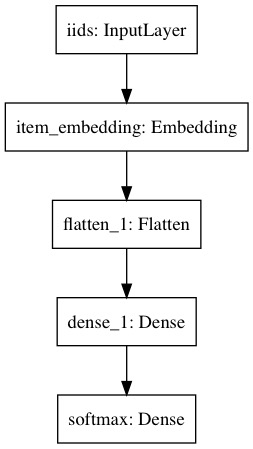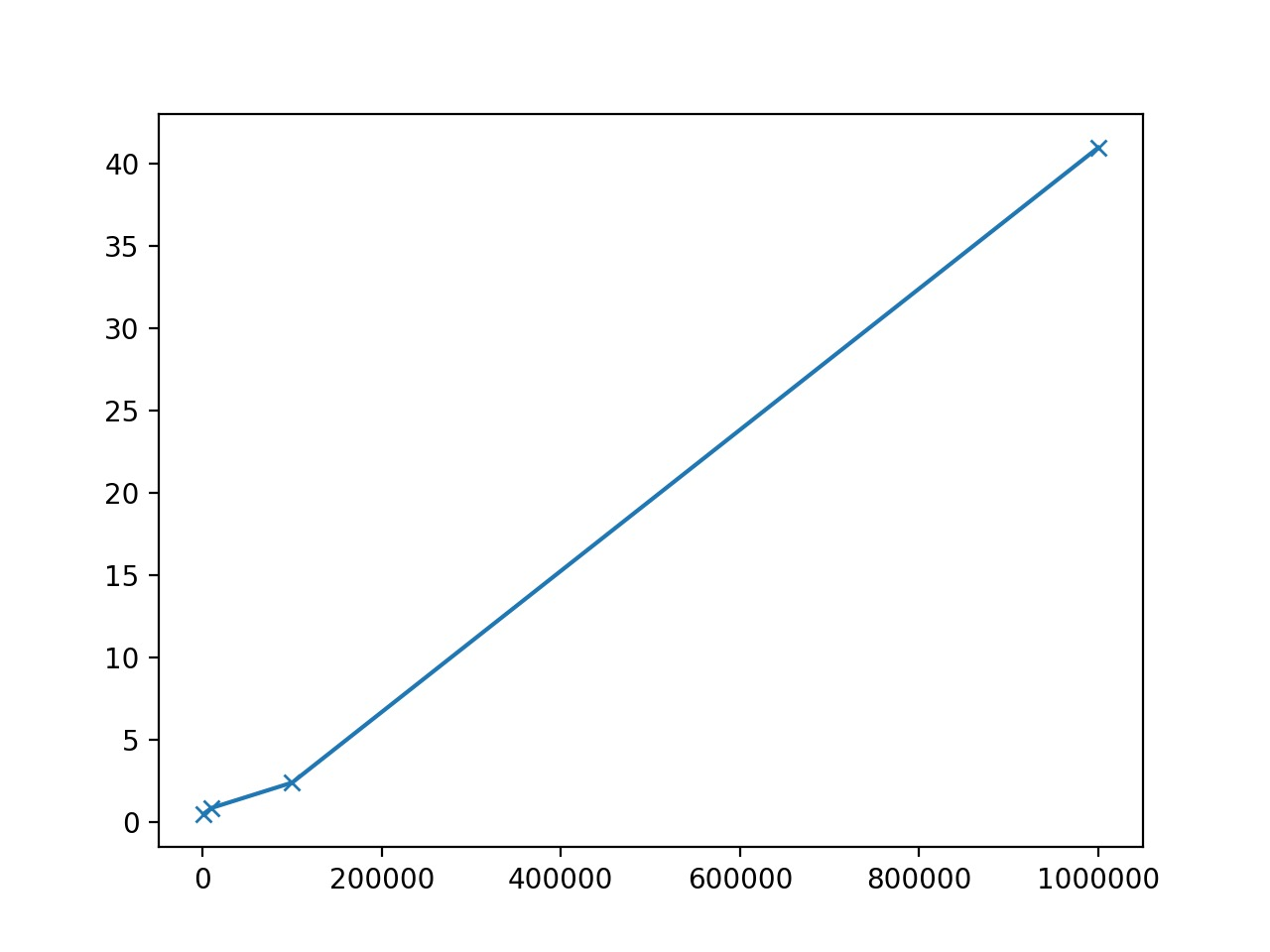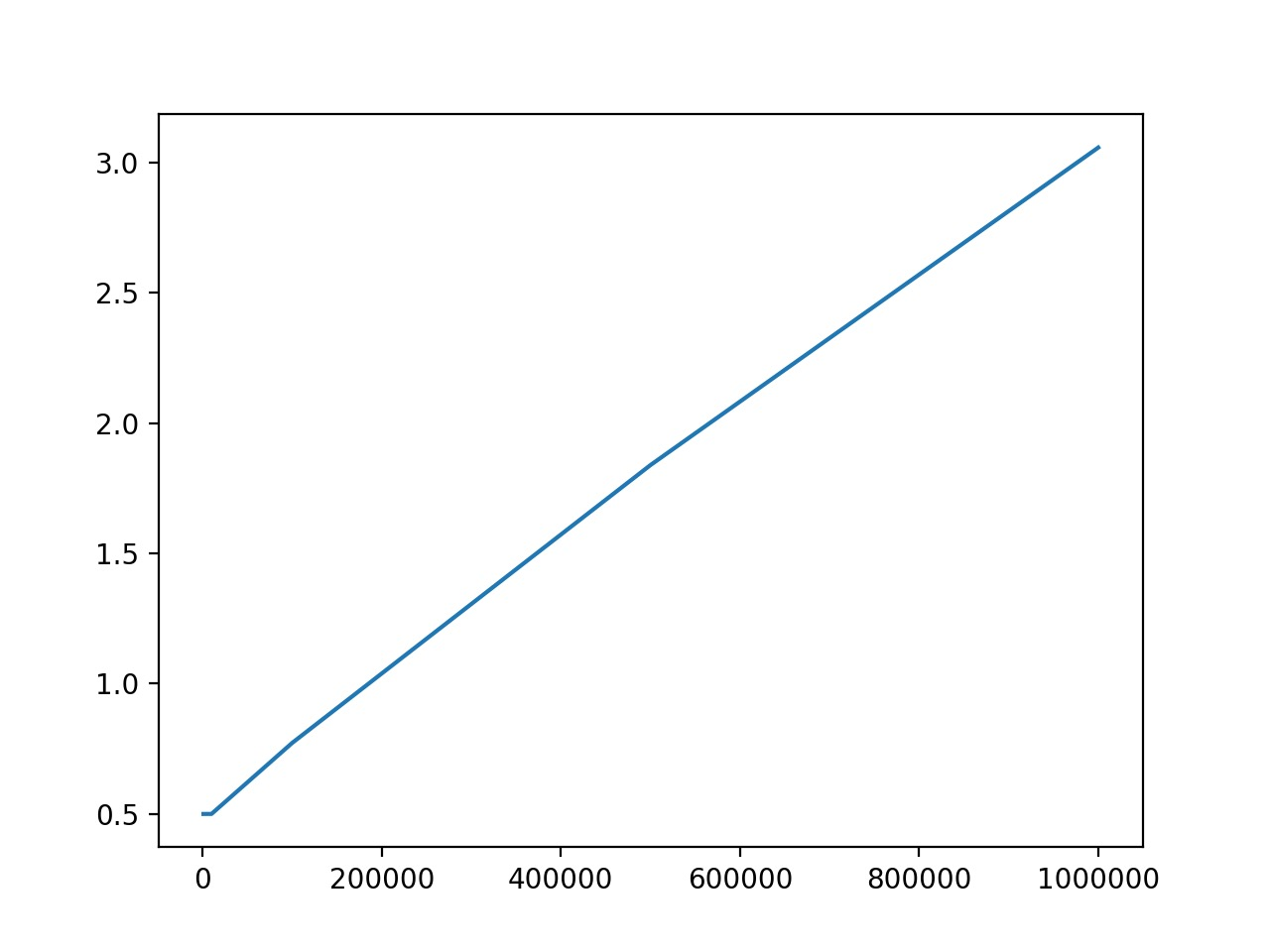# NCE: Noise-contrastive Estimation Loss

Alex Egg,

Candidate Sampling > Noise-contrastive Loss

## Candidate Sampling

Do you want to train a multiclass model with thousands or millions of output classes (for example, a language model with a large vocabulary)? Training with a full Softmax is slow in this case, since all of the classes are evaluated for every training example. Candidate Sampling training algorithms can speed up your train times by only considering a small randomly-chosen subset of “contrastive” classes (called candidates) for each batch of training examples. 1

A few examples of Candidate Sampling:

• Negative Sampling
• Sampled Softmax
• Hierarchal Softmax
• Noise-contrastive Estimation (NCE)

We will explore NCE in this post b/c it is implemented in Tensorflow along with Sampled Softmax.

## NCE

The full softmax will learn the fully-connected weight matrix by minimizing the normalized probability distribution using categorical cross entropy. NCE will learn the same weight matrix, but by a different learning objective. NCE does not try to estimate the probability of a item directly. Instead, it uses an auxiliary loss that also optimizes the goal of maximizing the probability of correct words.2

NCE learns a model to differentiate the target item/word from noise. By noise, we mean randomly sampled items/words instead of the whole vocabulary. We can thus reduce the problem of predicting the correct word to a binary classification task, where the model tries to distinguish positive, genuine data from noise samples. 2

You essentially train a noise/not-noise classifier instead of a full softmax classifier. We can now use logistic regression to minimize the log-likelihood using binary cross-entropy of our training examples against the noise.

### Tensorflow Example

Here, I’ll break down the NCE implementation.

#linear model (logistic) parameters
W = tf.get_variable('NCE_logisitic_multiclass_weights', [NUM_CLASSES, NDIM])
b = tf.get_variable('item_embed_bias', [NUM_CLASSES])

loss = tf.reduce_mean(
tf.nn.nce_loss(
weights=W,
biases=b,
labels=y,
inputs=h,
num_sampled=NUM_NEG_SAMPLES,
num_classes=NUM_CLASSES)
)


The parameters:

• Weights & biases: If you were learning a full softmax, it would introduce [NUM_CLASSES, dim] parameters. Since we are approximating a full softmax, we still need these parameters, so here they are.
• Labels & inputs: your training batch examples [batch_size, dim] and labels [batch_size, 1].
• Num_sampled: Number of negative samples. NCE paper suggests 24. I’ve seen 1000 work in non-word2vec scenarios.
• num_classes: typically your vocabulary size in word2vec. If this was Imagenet it would be 1000.

The function has two major steps:

1. Computes logits of true and sampled examples
2. Computes binary cross entropy loss on logits

Line by line explantation of the TF implementation:

1. labels_flat true labels
2. sampled_values Gets negative samples: Takes input the true classes in the batch and generates N classes not in that set. Which simply are the integers in the range 0:N that are not in true_classes (log_uniform_candidate_sampler)
3. all_ids = array_ops.concat([labels_flat, sampled], 0): contacts all positive and sampled item ids
4. all_w = embedding_ops.embedding_lookup(weights, all_ids): gets all the embeddings ready
5. true_w : true weights from embedding
6. sampled_w then they just get the sampled portion of all_w
7. sampled_logits = math_ops.matmul(inputs, sampled_w, transpose_b=True) then they do dot-product on neg samples - [batch_size, num_sampled]
8. all_b = embedding_ops.embedding_lookup(biases, all_ids): get all biases
9. true_b sampled_b : break into true and sampled parts
10. row_wise_dots = math_ops.multiply(array_ops.expand_dims(inputs, 1), array_ops.reshape(true_w, new_true_w_shape)): dot-product w/ input and pos weights
11. dots_as_matrix = array_ops.reshape(row_wise_dots: [batch_size, num_true] tensor of true_logits
12. true_logits = array_ops.reshape(_sum_rows(dots_as_matrix), [-1, num_true])
13. sampled_logits += sampled_b
14. out_labels = array_ops.concat([array_ops.ones_like(true_logits) ,array_ops.zeros_like(sampled_logits)], 1): these are the binary labels for the batch, 0s for negative samples.
15. out_logits = array_ops.concat([true_logits, sampled_logits], 1) concat true and samples
16. sampled_losses = sigmoid_cross_entropy_with_logits(labels=labels, logits=logits): Then it returns these logits, where it does x-entropy.

Then this is the loss for your whole model!: the dot product between the input $h$ and the positive item embeddings and dot product of the negative sampled items and the input. Add this binary cross entropy loss to your overall loss and it will in theory approximate the full softmax.

## Case Study: Extreme Multiclass w/ Full Softmax

Here is concrete demonstrating how a large softmax becomes computational intractable. Most image classification models (CNNs) based on the ImageNet dataset have 1000 wide softmax layers. You don’t usually see larger that, lets see why.

Consider the very simple neural net w/ and embedding on the input, 1 hidden layer and a softmax output layer:

from keras.layers import Input, Dense, Embedding, Flatten
from keras.models import Model
import numpy as np

def build(NUM_ITEMS, k):

iid = Input(shape=(1,), dtype='int32', name='iids')

item_embedding = Embedding(input_dim=NUM_ITEMS, output_dim=k, input_length=1, name="item_embedding")
selected_items = Flatten()(item_embedding(iid))

h1 = Dense(k//2, activation="relu")(selected_items)
sig = Dense(NUM_ITEMS, activation="softmax", name="softmax")(h1)

model = Model(inputs=[iid], outputs=sig)
return model


The input to the build function is the number of items in your embedding which is also the length of the softmax, similar to word2vec architectures. k is the dimension of the embedding hyper-parameter.Figure 1: Simple Feed Forward Net w/ 1 hidden layer and a softmax output layer

Let’s profile the training speed of this simple classifier for 1 training example across growing softmax sizes from 1000 to 1M:

import time
import matplotlib
import matplotlib.pyplot as plt

K=256
BATCH_SIZE=1
softmax_size=[1000, 10000, 100000, 1000000]
times=[]

for num_items in softmax_size:
print("\n\nSoftmax Width: ",num_items)
model = build(num_items, K)
model.summary()

t0 = time.time()

X=[np.random.rand(BATCH_SIZE,1)]
y=np.ones(BATCH_SIZE)
model.fit(x=X, y=y)

times.append(time.time() - t0)

plt.plot(softmax_size, times, '-x')
plt.show()

Dim 1,000 10,000 100,000 1,000,000
CPU 0.4 0.8 2.4 37.8
GPU* 0.5 0.5 0.7 3.0

Table 1: Training times for CPU and GPU across different softmax widths in seconds

*Tesla k80 11GB

Test Log:

Softmax Width:  1000
_________________________________________________________________
Layer (type)                 Output Shape              Param #
=================================================================
iids (InputLayer)            (None, 1)                 0
_________________________________________________________________
item_embedding (Embedding)   (None, 1, 256)            256000
_________________________________________________________________
flatten_1 (Flatten)          (None, 256)               0
_________________________________________________________________
dense_1 (Dense)              (None, 128)               32896
_________________________________________________________________
softmax (Dense)              (None, 1000)              129000
=================================================================
Total params: 417,896
Trainable params: 417,896
Non-trainable params: 0
_________________________________________________________________
Epoch 1/1
2018-09-30 00:40:47.678912: I tensorflow/core/platform/cpu_feature_guard.cc:141] Your CPU supports instructions that this TensorFlow binary was not compiled to use: AVX2 FMA

1/1 [==============================] - 0s 244ms/step - loss: 6.9035

Softmax Width:  10000
_________________________________________________________________
Layer (type)                 Output Shape              Param #
=================================================================
iids (InputLayer)            (None, 1)                 0
_________________________________________________________________
item_embedding (Embedding)   (None, 1, 256)            2560000
_________________________________________________________________
flatten_2 (Flatten)          (None, 256)               0
_________________________________________________________________
dense_2 (Dense)              (None, 128)               32896
_________________________________________________________________
softmax (Dense)              (None, 10000)             1290000
=================================================================
Total params: 3,882,896
Trainable params: 3,882,896
Non-trainable params: 0
_________________________________________________________________
Epoch 1/1

1/1 [==============================] - 1s 569ms/step - loss: 9.2097

Softmax Width:  100000
_________________________________________________________________
Layer (type)                 Output Shape              Param #
=================================================================
iids (InputLayer)            (None, 1)                 0
_________________________________________________________________
item_embedding (Embedding)   (None, 1, 256)            25600000
_________________________________________________________________
flatten_3 (Flatten)          (None, 256)               0
_________________________________________________________________
dense_3 (Dense)              (None, 128)               32896
_________________________________________________________________
softmax (Dense)              (None, 100000)            12900000
=================================================================
Total params: 38,532,896
Trainable params: 38,532,896
Non-trainable params: 0
_________________________________________________________________
Epoch 1/1

1/1 [==============================] - 2s 2s/step - loss: 11.5137

Softmax Width:  1000000
_________________________________________________________________
Layer (type)                 Output Shape              Param #
=================================================================
iids (InputLayer)            (None, 1)                 0
_________________________________________________________________
item_embedding (Embedding)   (None, 1, 256)            256000000
_________________________________________________________________
flatten_4 (Flatten)          (None, 256)               0
_________________________________________________________________
dense_4 (Dense)              (None, 128)               32896
_________________________________________________________________
softmax (Dense)              (None, 1000000)           129000000
=================================================================
Total params: 385,032,896
Trainable params: 385,032,896
Non-trainable params: 0
_________________________________________________________________
Epoch 1/1

1/1 [==============================] - 41s 41s/step - loss: 13.8165Figure 2: CPU train times

As you can see the 1M wide softmax took 41 seconds to train just 1 example and the 100k took 2 seconds.Figure 3: GPU Train times

As you can see, 1 training example taking a half a around a second to process does not scale well. Granted we can process in batches, but the overhead should still be apparent. Another variable is the complexity of your network, most will not be as simple as this one.

## Keras NCE Implementation

Now let’s try the same model above, but let’s replace the full Softmax w/ an approximate softmax implemented as NCE.

Full code is here: https://github.com/eggie5/NCE-loss

To do this w/ keras it’s a tiny-bit hackery. You have to use the Layer’s API, as opposed to the Loss API, and pass in your labels as inputs:

import keras
from keras.layers import Input, Dense, Embedding, Flatten
import tensorflow as tf
import keras.backend as K

class NCE(keras.layers.Layer):
def __init__(self, num_classes, neg_samples=100, **kwargs):

self.num_classes = num_classes
self.neg_samples = neg_samples

super(NCE, self).__init__(**kwargs)

# keras Layer interface
def build(self, input_shape):

name="approx_softmax_weights",
shape=(self.num_classes, input_shape),
initializer="glorot_normal",
)

name="approx_softmax_biases", shape=(self.num_classes,), initializer="zeros"
)

# keras
super(NCE, self).build(input_shape)

# keras Layer interface
def call(self, x):
predictions, targets = x

# tensorflow
loss = tf.nn.nce_loss(
self.W, self.b, targets, predictions, self.neg_samples, self.num_classes
)

# keras

logits = K.dot(predictions, K.transpose(self.W))

return logits

# keras Layer interface
def compute_output_shape(self, input_shape):
return 1


As you can see, like the normal Keras Softmax layer we define our weight matrix and biases, however, the special thing here is that we add a loss to the Keras runtime. The loss is the returned value from the TF implementation of NCE. Here is the new model w/ the NCE loss:

from keras.layers import Input, Dense, Embedding, Flatten,
from keras.models import Model
import keras.backend as K
import numpy as np
from nce import NCE

def build(NUM_ITEMS, num_users, k):

iid = Input(shape=(1,), dtype="int32", name="iids")
targets = Input(shape=(1,), dtype="int32", name="target_ids")

item_embedding = Embedding(
input_dim=NUM_ITEMS, output_dim=k, input_length=1, name="item_embedding"
)
selected_items = Flatten()(item_embedding(iid))

h1 = Dense(k // 2, activation="relu", name="hidden")(selected_items)
sig = Dense(NUM_ITEMS, activation="softmax", name="softmax")(h1)

sm_logits = NCE(num_users, name="nce")([h1, targets])

model = Model(inputs=[iid, targets], outputs=[sm_logits])
return model

K = 10
SAMPLE_SIZE = 10000
num_items = 10000
NUM_USERS = 1000000

model = build(num_items, NUM_USERS, K)
model.summary()

x = np.random.random_integers(num_items - 1, size=SAMPLE_SIZE)
y = np.ones(SAMPLE_SIZE)
X = [x, y]
print(x.shape, y.shape)

model.fit(x=X, batch_size=100, epochs=1)


Here is the output:

Using TensorFlow backend.
__________________________________________________________________________________________________
Layer (type)                    Output Shape         Param #     Connected to
==================================================================================================
iids (InputLayer)               (None, 1)            0
__________________________________________________________________________________________________
item_embedding (Embedding)      (None, 1, 10)        100000      iids
__________________________________________________________________________________________________
flatten_1 (Flatten)             (None, 10)           0           item_embedding
__________________________________________________________________________________________________
hidden (Dense)                  (None, 5)            55          flatten_1
__________________________________________________________________________________________________
target_ids (InputLayer)         (None, 1)            0
__________________________________________________________________________________________________
nce (NCE)                       1                    6000000     hidden
target_ids
==================================================================================================
Total params: 6,100,055
Trainable params: 6,100,055
Non-trainable params: 0
__________________________________________________________________________________________________
(10000,) (10000,)
Epoch 1/1
10000/10000 [==============================] - 7s 704us/step - loss: 578.2593
(10, 1000000)


As you can see we our NCE layer made a [1e6, 10] weight matrix and a [1e6] bias array for a total of 6e6 parameters which has an equal amount of expressive power as a full softmax for 1M items!

Also, as you can see I trained over 10k items on a CPU in 7s, which is 1.4k examples/second! Much faster than the full softmax!

1. https://www.tensorflow.org/api_guides/python/nn#Candidate_Sampling

2. http://ruder.io/word-embeddings-softmax/  2